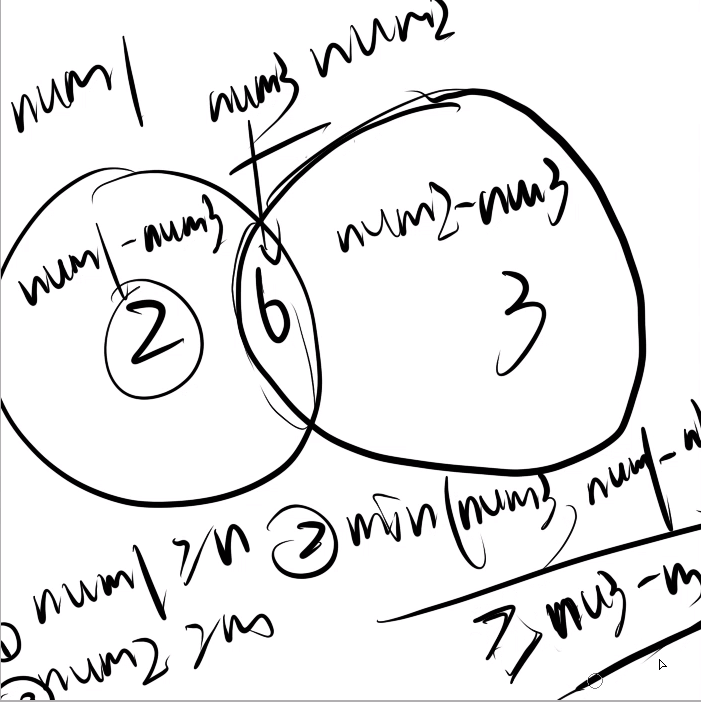## C. Block Towers(二分)

C. Block Towers
time limit per test
2 seconds
memory limit per test
256 megabytes
input
standard input
output
standard output

Students in a class are making towers of blocks. Each student makes a (non-zero) tower by stacking pieces lengthwise on top of each other. n of the students use pieces made of two blocks and m of the students use pieces made of three blocks.

The students don’t want to use too many blocks, but they also want to be unique, so no two students’ towers may contain the same number of blocks. Find the minimum height necessary for the tallest of the students' towers.

Input

The first line of the input contains two space-separated integers n and m (0 ≤ n, m ≤ 1 000 000n + m > 0) — the number of students using two-block pieces and the number of students using three-block pieces, respectively.

Output

Print a single integer, denoting the minimum possible height of the tallest tower.

Examples
input
Copy
```1 3
```
output
Copy
```9
```
input
Copy
```3 2
```
output
Copy
```8
```
input
Copy
```5 0
```
output
Copy
```10
```
Note

In the first case, the student using two-block pieces can make a tower of height 4, and the students using three-block pieces can make towers of height 36, and 9 blocks. The tallest tower has a height of 9 blocks.

In the second case, the students can make towers of heights 24, and 8 with two-block pieces and towers of heights 3 and 6 with three-block pieces, for a maximum height of 8 blocks.num1:2的倍数

num2:3的倍数

num3:6的倍数 然后可以推出上图的三个限定条件

ac代码

```#include<bits/stdc++.h>

using namespace std;

int n,m;

bool check(int x)
{
int num1 = x/2;
int num2 = x/3;
int num3 = x/6;
if(num1 < n)
return false;
if(num2 < m)
return false;
if(min(num3,num1-n)<m-(num2-num3) )
return false;

return true;

}

int main()
{
cin>>n>>m;
int l = 0 ,r = 1e7 ,ans = 0;
while(l <= r)
{
int mid = (l+r)/2;
if(check(mid))
{
ans = mid;
r = mid-1;
}
else
l = mid+1;
}
cout<<ans<<endl;
}
```